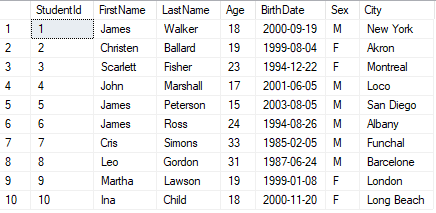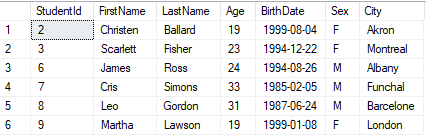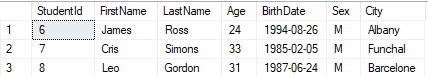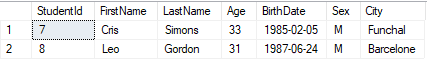WHERE Clause in SQL SERVER

## WHERE Definition

WHERE clause in SQL SERVER enables you to apply one or multiple conditions before retrieving data from one or multiple tables.

## WHERE Usage

We use the WHERE clause by specifying the conditions to be met for the rows to be returned.

``SELECT column1, column2,... FROM table_name WHERE Condition``

In order to apply multiple conditions in a WHERE query, we use the following logical operators : AND, OR

## AND Operator

The AND operator signifies that all conditions must be true for a row in order to return it.

``SELECT column1, column2,... FROM table_name WHERE condition1 AND condition2, ...``

## OR Operator

The OR operator signifies that at least one condition must be true for a row in order to return it.

``SELECT column1, column2,... FROM table_name WHERE condition1 OR condition2, ...``

## WHERE Examples

For our examples, we will use a student table containing 10 rows of data.To show all students older than 18 years old :

``SELECT * FROM Student WHERE Age > 18``To show all male students older than 18 years old :

``SELECT * FROM Student WHERE Sex = 'M' AND Age > 18``To show all students that are either born in 1985 or born in Barcelone :

``SELECT * FROM Student WHERE YEAR(BirthDate) = 1985 OR City = 'Barcelone'``